# ISNONTEXT Function Examples – Excel, VBA, & Google Sheets

This tutorial demonstrates how to use the Excel ISNONTEXT Function in Excel to test if a cell is not text.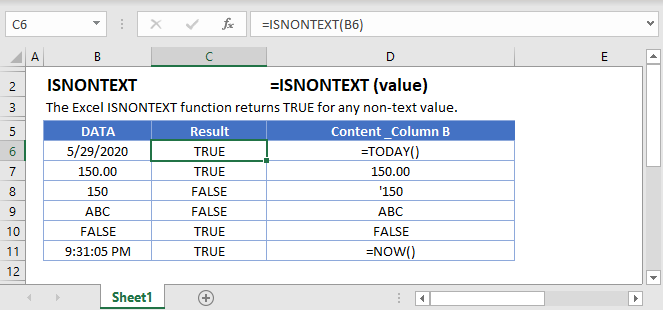## ISNONTEXT Function Overview

The ISNONTEXT Function Test if cell is not text (blank cells are not text). Returns TRUE or FALSE.

To use the ISNONTEXT Excel Worksheet Function, select a cell and type: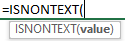(Notice how the formula inputs appear)

### ISNONTEXT Function Syntax and Inputs:

`=ISNONTEXT(VALUE)`

value – The test value

## How to use the ISNONTEXT Function

The ISNONTEXT Function tests if a cell is not text. If the cell is not text it returns TRUE, otherwise FALSE.

`=ISNONTEXT(A2)`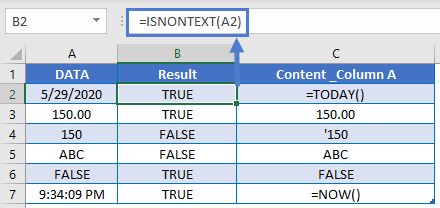### Numbers Stored as Text

When working with Excel, you might encounter numbers stored as text. Excel treats these numbers stored as text as text and not numbers, which can present problems for your formulas. One option is to use the VALUE Function to convert a number stored as text to a number.

Instead, however, you might simply want to test if a cell value is not text. Notice how the ISNONTEXT function returns FALSE if it encounters a number stored as text.

`=ISNONTEXT(A2)`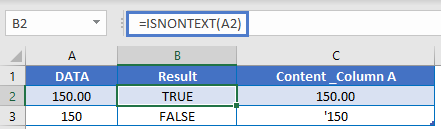### If Is Nontext

Usually, after performing a logical test, you will want to do something based on the result of that test. The IF Function can be used to perform different actions if a statement is evaluated as TRUE or FALSE. Let’s use it with the ISNONTEXT Function.

`=IF(ISNONTEXT(A2),"non text", "text")`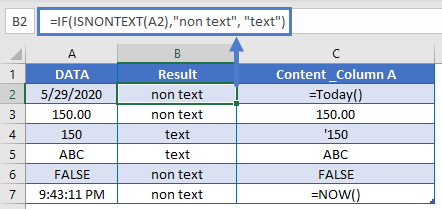### ISTEXT Function

The ISNONTEXT Function is the opposite of the ISTEXT Function. Our tutorial on the ISTEXT Function contains many more examples for working with cells that may or may not contain text.

### Other Logical Functions

Excel / Google Sheets contain many other logical functions to perform other logical tests. Here is a list:

IF / IS Functions
iferror
iserror
isna
iserr
isblank
isnumber
istext
isnontext
isformula
islogical
isref
iseven
isodd

The ISNONTEXT Function works exactly the same in Google Sheets as in Excel: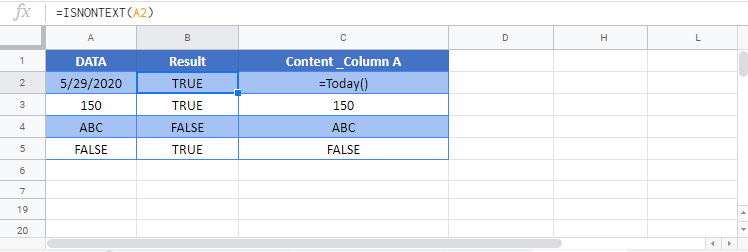## ISNONTEXT Examples in VBA

You can also use the ISNONTEXT function in VBA. Type:
`application.worksheetfunction.isnontext(value)`
For the function arguments (value, etc.), you can either enter them directly into the function, or define variables to use instead.